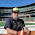### Physics 9B Practice Final Problem #1

Water (density 103 kg/m3) is being siphoned out of a very large tank. The pipe extends a distance 3 m into the water and rises to a height 4 m above the water before dropping a distance 8 m. On the surface of the water, the pressure is 105 N/m2.
a) What is the flow speed in the pipe?
b) Is there a limit on how high the siphon top can rise above the surface of the water and still work? Explain. If so, what is the limit?
Question so kindly provided by Joe Kiskis

Part A
To solve this, simply apply Bernoulli's principle:
• P + ρgy + 1/2 ρv2 = C
• P - pressure
• ρ - Density
• g - acceleration due to gravity
• y - height from arbitrary reference point
• v - velocity of the fluid
• C - arbitrary constant
We're trying to find the velocity of the fluid in the tube, so we can choose any point in the tube. We also need another point to calculate C; the surface of the water should do, since we know everything about it.
• 105N/m2 + 103 kg/m3*9.8m/s2*0m + 1/2*103 kg/m3*(0m/s)2 = 105N/m2
• We can arbitrarily set the origin for y as the surface of the water, but really any other point would do, just not as easily.
• We can assume the surface of the water isn't moving, since this is a lousy lower division physics class.
• C = 105N/m2
Now that we know C, we can plug it into the equation anywhere else. The easiest place would be at the mouth of the tube, since at that point it equalizes pressure with air, who's pressure was given.
• 105N/m2 + 103 kg/m3*9.8m/s2*(-4m) + 1/2*103 kg/m3*v2 = 105N/m2
• y = -4m since the tube goes up 4m, then down 8m.
• Now just solve for v
• v = 8.85 m/s
Part B
There is an upper limit to how high the siphon can operate, since it's the surface air pressure that is pushing the water up the tube. Once the weight of the water exceeds the air pressure, the water can't raise any farther. Above this upper limit, the inside of the tube is a vacuum, so to find this limit, set P=0. At the same time, energy has to be conserved, and we know the water is moving through the tube at 8.85 m/s, so we need to factor the kinetic energy into the use of Bernoulli's principle When the siphon fails, the water stops moving, which means that it's only the height of the tube that matters:
• 0 + ρgy + 0 = C
• Solve for y
• y = C / ρg
• y = 9.75 m 10.2 m
Note: The use of KE in this equation originally seemed iffy to me. Consider this: the water in the tank is compressed to 105 N/m2 by the air. The water then needs to be accelerated into the tube, which will translate some of this pressure into KE, and will lower the maximum height from the stationary column of water height of 10.2m.
Note: I was all kinds of confused on this problem. Thankfully, the professor was kind enough to drop into the classes chat room and point out all my failures in logic.

Now the real question is what happens between 9.75m and 10.2m? On the one hand, the siphon still works as long as the water is moving, but we've already calculated how fast the water has to exit the tube to conserve the lost potential energy of dropping 4m. How can the water slow down at the top of the tube?

1.Well, the maximum height for pumping water using a suction pump is 32 feet, so based on empirical evidence I don't think you need to factor in the KE. Your example is at standard pressure, so empirical evidence is pretty accurate. The Also, at the failure point, water won't be flowing, so there won't be any KE. Amright? (It's been a few years since I've done any physics)

2.Citation for the 32 foot comment:

P. 73 Burke, James. "Connections" 1978.

3.Yeah, but at the same time, we know the total energy when it enters and exits the tube, so how can the fluid slow down in the middle of the (assumed uniform) tube, but speed up again? So I can really argue it either way.

4.Clearly, we need a 4 story building and a long tube. :)

5.An an incompressible non-viscous fluid.

6.Nope, prompt specifies water, so water we shall test with! Although arguably water is pretty close. Acetone has a lower viscosity and is incompressible...

Also, the waters not slowing down, it's the height above which the siphon just wouldn't work. Thus, no KE.

7.Don't you love how he didn't bother to specify the implicit assumptions?
The water may not be slowing down, but it is speeding up after being compressed by air as it travels into the tube, which translates into lost pressure.

8.So in the end, 10.2m was right, and I was just over-thinking the problem.